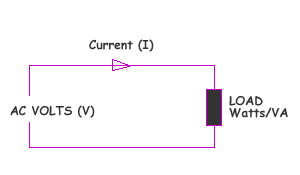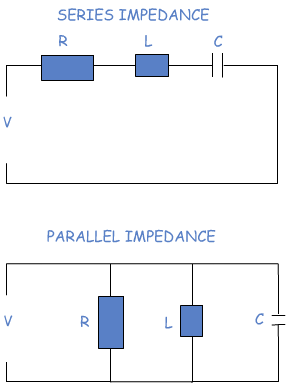ABOUT | DISCLAIMER | FEEDBACK | PRIVACY

 ELECTRIC CALCULATIONS

 AC CURRENT AND POWER CALCULATIONS SELECTCurrent CalculationPower Calculation   Enter Power or Current:   Enter Volts per phase:      Power Factor(Optional):  Values 0-1         Result:NOTE: Negligible circuit (cable) impedance is assumed.

 IMPEDANCE CALCULATION VOLTAGE (V):     Volts FREQUENCY (F):  Hz RESISTANCE (R):   Ω * INDUCTANCE (L): mH  * CAPACITANCE (C):   ï¿½F  *                                       RESULTS:  * Note: For calculation purpose, zero (0) value for these components, are interpreted to mean "not in circuit".POWER FACTOR CALCULATION Generally, it is desired to have a power factor as close as possible to unity(1). At this value the current will be the lowest, and the Volt-amperes demand will be the lowest. The Effects of Power Factor On Volt Ampere(s) for one phase. Enter Load: Watts Enter Desired Volt-Amperes:  Volt Amps must be => than Load entered.      Result:  Click "Demo" below to see Graphical Demonstration Of Volt Amperes variation with power factor: